# Q-Meter | Working & Applications

Q-meter is an instrument designed for measuring Q-factor of a coil as well as for the measurement of inductance, capacitance, and resistance of an electric circuit at radio frequency. Q-meter is also known as RLC meter.

What is Q-factor of the coil?

The ratio of the inductive reactance to the effective resistance of the coil is called the Q-factor of the coil.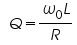## Circuit Diagram of Q-meter

The Q-meter operates on the principle of series resonance i.e. under the resonant condition of an AC series circuit voltage across the capacitor is equal to the applied voltage times of Q-factor of the circuit. If the applied voltage across the circuit is kept-constant then voltmeter connected across the capacitor can be calibrated to indicate Q-factor value directly.

The practical circuit for measurement of Q-factor shown in Figure 1. The oscillator is set to the desired frequency and then the tuning capacitor (C) is adjusted for maximum value Vc. The input voltage V is kept constant then the voltage across the capacitor (Vc) is calibrated to read the value of Q-factor value directly. The measured value of Q-factor value is defined for the whole circuit, not of the coil alone. There are the errors caused due to shunt resistance and distributed capacitance of the circuit.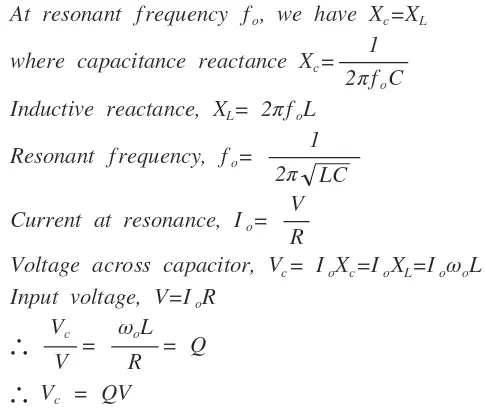Correction for shunt resistance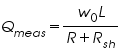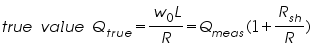Correction for distributed capacitance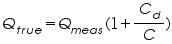where, Cd = distributed capacitor

C = tuning capacitance

Measurement of self Capacitance

The value of inductance is given by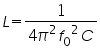the values of f0 and C are known and therefore the value of inductance may be calculated.

Measurement of Effective Resistance

The value of effective resistance may be computed from the relation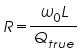Measurement of Self-Capacitance

The self-capacitance of the coil is measured by making two measurements at different frequencies. The capacitor is set to a high value and the circuit is resonated by adjustment of the oscillator frequency. The Resonance is indicated by the circuit Q-meter. Let the value of tuning capacitor be C1 and that of frequency be f1 under this condition. Therefore,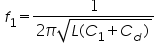The frequency is now increased to twice its initial value and the circuit is resonated again this time with the help of the tuning capacitor. Let the values of tuning capacitor be C2 and that of frequency be f2 under this condition. Therefore,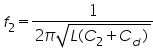Now, f2=2*f1

Then distributed capacitance, Cd is given by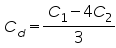## Applications of Q-Meter

The Q meter can be used for many purposes.

1. As the name implies, it can measure Q and is generally used to check the Q factor of inductors.
2. It is used to measure self-capacitance of the coil.
3. The characteristic impedance of a transmission line can be measured using series substitution method.
4. It is used for the measurement of bandwidth.
5. Q-meter is used for the measurement of capacitance. The capacitor sample is resonated with a selected inductor by adjusting the source frequency and by using the tuning capacitor set to a low value on its calibrated scale.

### 1 thought on “Q-Meter | Working & Applications”

1.it is very useful content…………. thank you guys

error: Content is protected !!